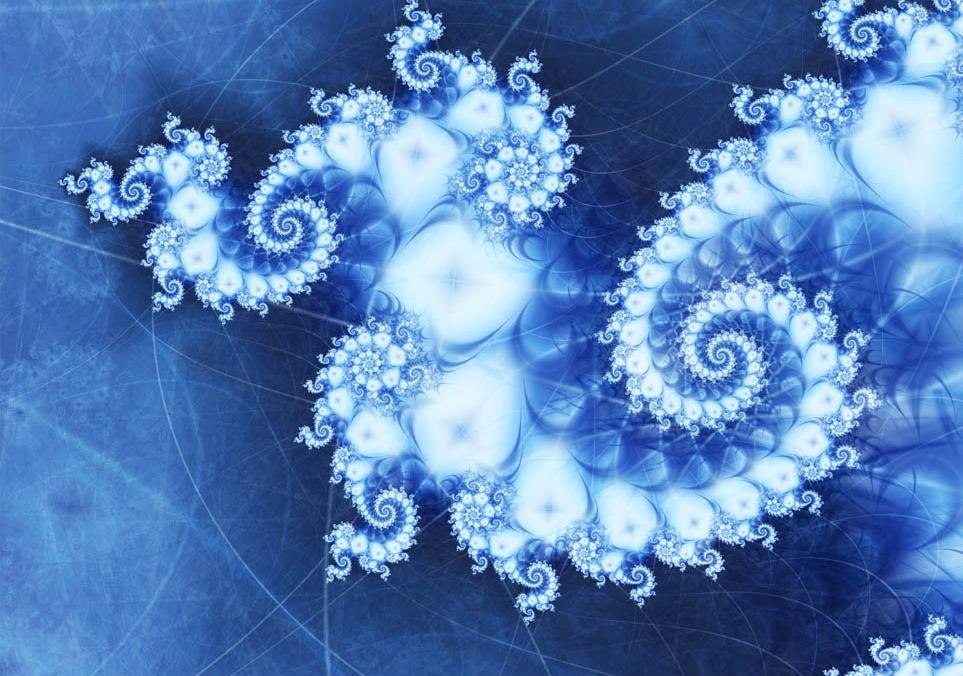# Fractal ExplorationConsider an image, fully-detailed, perfectly square, but initially represented as but a single, uniform pixel, the average of all of its contents. Naturally, one would wish to see more - more details, more pixels, more depth, but receiving all of this information instantaneously, perfectly, can be so... boring.

Instead, let us explore the image, piece by piece, quadrant by quadrant. The first pixel is given to us for free, and to see more, we must explore it - digging deeper, one 'pixel' may be split into four, each being the average of the top-left, top-right, bottom-left, and bottom-right subspaces it covers respectively, all equal in size. Each of these, we may then, in turn, explore, revealing more and more detail as we go, until the pixels revealed are, in fact, pixels, yielding no further detail than themselves.

In this way, what was once a fixed, linear journey - row by row, column by column - has become something much richer! There are many different ways one could reveal the full landscape, many different paths one could take to reveal each detail. For a $1 \times 1$ image, or a $2 \times 2$ image, there is but one way forward, but for a $4 \times 4$ image, there are $24$ ways to reveal everything! One touch on the center to reveal $4$ distinct $2 \times 2$ 'pixels', which can, of course, be explored in $4!$ different orders to reveal the entire contents of the landscape. However, this number gets much richer, very quickly.

A $8 \times 8$ image can be fully explored in $3892643213082624$ ways. That's right, over $3$ quadrillion paths can be taken.

Let $N$ be the number of ways a $512 \times 512$ landscape can be fully explored. The number $N$ has over three-hundred thousand decimal digits, so calculating it is no mere pencil-and-paper operation.

What is the digit-sum of $N$?

Inspired by this class of interactive animation.

(Took me a while to find a link! Hard to search for, these were somewhat popular a couple years ago.)